WHERE-Expression Processing

# Processing a Segment of Data That Is Conditionally Selected

When you conditionally select a subset of observations with a WHERE expression, you can also segment that subset by applying FIRSTOBS=, OBS= or both processing (as data set options and system options). When used with a WHERE expression,

• FIRSTOBS= specifies the observation number within the subset of data selected by the WHERE expression to begin processing.

• OBS= specifies when to stop processing observations from the subset of data selected by the WHERE expression.

When used with a WHERE expression, the values specified for OBS= and FIRSTOBS= are not the physical observation number in the data set, but a logical number in the subset. For example, obs=3 does not mean the third observation number in the data set; instead, it means the third observation in the subset of data selected by the WHERE expression.

Applying OBS= and FIRSTOBS= processing to a subset of data is supported for the WHERE statement, WHERE= data set option, and WHERE clause in the SQL procedure.

If you are processing a SAS view that is a view of another view (nested views), applying OBS= and FIRSTOBS= to a subset of data could produce unexpected results. For nested views, OBS= and FIRSTOBS= processing is applied to each SAS view, starting with the root (lowest-level) view, and then filtering observations for each SAS view. The result could be that no observations meet the subset and segment criteria. See Processing a SAS View.

The following SAS program illustrates how to specify a condition to subset data, and how to specify a segment of the subset of data to process.

```data A; 1
do I=1 to 100;
X=I + 1;
output;
end;
run;

proc print data=work.a (firstobs=2 3  obs=4 4  ;
where I > 90; 2
run;```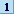The DATA step creates a data set named WORK.A containing 100 observations and two variables: I and X.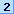The WHERE expression I > 90 tells SAS to process only the observations that meet the specified condition, which results in the subset of observations 91 through 100.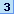The FIRSTOBS= data set option tells SAS to begin processing with the 2nd observation in the subset of data, which is observation 92.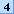The OBS= data set option tells SAS to stop processing when it reaches the 4th observation in the subset of data, which is observation 94.

The result of PROC PRINT is observations 92, 93, and 94.

The following SAS program creates a data set, a SAS view for the data set, then a second SAS view that subsets data from the first SAS view. Both a WHERE statement and the OBS= system option are used.

```data a; 1
do I=1 to 100;
X=I + 1;
output;
end;
run;

data viewa/view=viewa; 2
set a;
Z = X+1;
run;

data viewb/view=viewb; 3
set viewa;
where I > 90;
run;

options obs=3; 4

proc print data=work.viewb; 5
run;```The first DATA step creates a data set named WORK.A, which contains 100 observations and two variables: I and X.The second DATA step creates a SAS view named WORK.VIEWA containing 100 observations and three variables: I, X (from data set WORK.A), and Z (assigned in this DATA step).The third DATA step creates a SAS view named WORK.VIEWB and subsets the data with a WHERE statement, which results in the view accessing ten observations.The OBS= system option applies to the previous SET VIEWA statement, which tells SAS to stop processing when it reaches the 3rd observation in the subset of data being processed.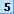When SAS processes the PRINT procedure, the following occurs: First, SAS applies obs=3 to WORK.VIEWA, which stops processing at the 3rd observation. Next, SAS applies the condition I > 90 to the three observations being processed. None of the observations meet the criteria. PROC PRINT results in no observations.

To prevent the potential of unexpected results, you can specify obs=max when creating WORK.VIEWA to force SAS to read all the observations in the root (lowest-level) view:

```data viewa/view=viewa;
set a (obs=max);
Z = X+1;
run;```

The PRINT procedure processes observations 91, 92, and 93.Previous Page | Next Page | Top of Page